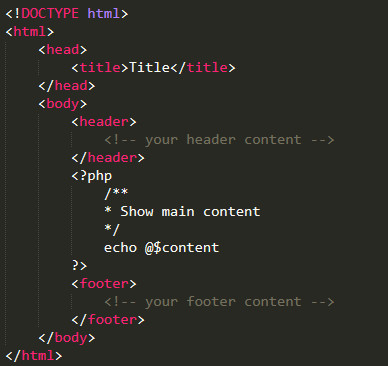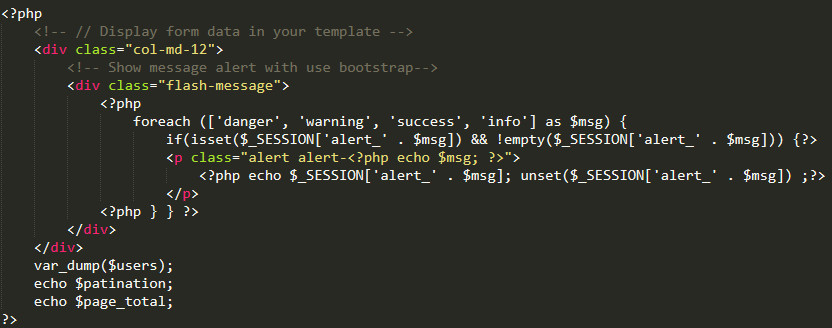# PHP – MVC CRUD and Connect to MySQL using PDO

in PHP MySQL

Basic knowledge is always very important, in this article I will introduce to you how to use the PHP – MVC model, using PDO to connect to MySQL.

What is PDO?

It is an acronym for “PHP Data Objects”. Until now, the functions used were divided according to the type of database.

For example:

– When connecting to MySQL, I used to connect with the “mysql_connect” function,
– In the case of PostgreSQL, it was connected with the “pg_connect” function.

There is no problem if the database is fixed, but if you change or port to a different database in the future, you have to rewrite everything.

But if you use the PDO class, the same function can be used regardless of which database is used, so if you change the database in the future, you only need to change the parameters you specified when you created the PDO class (it seems that there are some differences depending on the database…)

Let’s learn how to connect to a database using the PDO class in this article.

We will proceed on the premise of the following conditions:

* PHP is PHP 5.3.6 or higher
* Database is MySQL
* Character encoding is UTF-8## I. Connect to DB with PDO

I assume for example you have a DB with the following information:

Name: YOUR_DB_NAME,
Port: 3306
Table: users (id, first_name, last_name, age)

Create a Class DB and use PDO to connect to your Database as follows. Specifies the character encoding. Note that it is utf8, not UTF-8

Create the connection.php file on your root directory:

connection.php

class DB
{
/**
* Static variable DB Name
*/
public function config()
{
return [
‘db_name’ => ‘YOUR_DB_NAME’,
];
}

/**
* Connect to Database
*/
public static function getInstance()
{
\$db = new DB();
\$db_name = \$db->config()[‘db_name’];

try {
\$pdo = new PDO(‘mysql:host=localhost; port=3306; dbname=’ . \$db_name, ‘root’, ‘123456’);
\$pdo->exec(“SET NAMES ‘utf8′”);
} catch (PDOException \$ex) {
\$pdo = \$ex->getMessage();
}

return \$pdo;
}
}

Using the above PDO statement, we were able to connect to the DB successfully.

When executing SQL statements with PDO, the following two types of methods can be used.
– query
– prepare

Next, let’s take a closer look at each method.

In addition, we create Controller, Model, View in turn to get data through PDO

## II. Controller, Model, View

1. Create the IndexController.php on /root/controllers folder

controllers/UsersController.php

require_once (‘controllers/BaseController.php’);
require_once (‘models/user.php’);

class UsersController extends BaseController
{
/**
* Construct
*/
public function __construct()
{
\$this->folder = ‘users’;

// Call perpage of pagination setting
\$this->status_success = 1;
\$this->status_error = 0;
\$this->per_page = 10;

\$this->save_success = ‘Data has been saved successfuly’;
\$this->save_error = ‘Data has been saved failed’;
\$this->delete_success = ‘Data has been deleted successfuly’;
\$this->delete_error = Data has been deleted failed;
}

/**
* Index
*/
public function index()
{
// Set default perpage
\$per_page = \$this->per_page

[\$users, \$pagination, \$page_total] = User::all(\$per_page);

\$data = [‘users’ => \$users, ‘pagination’ => \$pagination, ‘per_page’ => \$per_page, ‘page_total’ => \$page_total];

\$this->render(‘index’, \$data);
}
}

2. Create the BaseController.php on /root/controllers folder

controllers/BaseController.php

/**
* Base Controller
*/
class BaseController
{
// variable folder name in to access views folder
protected \$folder;

/**
* Show result to views
*/
function render(\$file, \$data = array()) {
// Check exist current file
\$view_file = ‘views/’ . \$this->folder . ‘/’ . \$file . ‘.php’;

if (is_file(\$view_file)) {
// if exist file, create variable and add to call function
extract(\$data);

// Save data and show with variable to views
ob_start();
require_once(\$view_file);
\$content = ob_get_clean();

// use common template
require_once(‘views/layouts.php’);
} else {
// if file not exist, show error page
}
}
}

3. Next, create the file user.php in /root/models folder

models/user.php

/**
* User Model
*/
Class User
{
/**
* List all
*/
public function all(\$per_page)
{
\$db = DB::getInstance();
if (isset(\$_GET[‘page’])) {
\$page = (int)\$_GET[‘page’];
} else {
\$page = 1;
}

if (\$page > 1) {
\$start = (\$page * \$per_page) – \$per_page;
} else {
\$start = 0;
}

// query get pagination, page_total
\$page_num = \$db->prepare(“SELECT count(*) FROM users WHERE ‘last_name’ = ? AND age = ?”);
\$page_num->execute();
\$page_total = \$page_num->fetchColumn();
\$pagination = ceil(\$page_total / \$per_page);

// query get list data
// your query is select all of them (SELECT * FROM users LIMIT {\$start}, \$per_page)
// or add conditions with last_name and age value

\$last_name = ‘John’;
\$age = 20;
\$request = \$db->prepare(“SELECT * FROM users WHERE ‘last_name’ = ? AND age = ? LIMIT {\$start}, \$per_page “);

\$lists = [];
if (\$request->execute([\$last_name, \$age]) {
foreach (\$request->fetchAll() as \$item) {
\$list = [];
\$list[‘first_name’] = \$item[‘first_name’];
\$list[‘last_name’] = \$item[‘last_name’];
\$list[‘age’] = \$item[‘age’];

\$lists[] = \$list;
}
}

return [\$lists, \$pagination, \$page_total];
}
}

// Pattern 1
\$request = \$db->prepare(“SELECT * FROM users WHERE ‘last_name’ = ? AND age = ?”);
\$request->execute([‘John’, 20]);

// Pattern 2
\$request = \$dbh->prepare(“SELECT * FROM users WHERE last_name = :last_name AND age = :age”);
\$request->bindValue(‘:last_name’, ‘John’);
\$request->bindValue(‘:age’, 20, PDO::PARAM_INT);
\$request->execute();

4. Next, create the view file in /root/views/users folder
views/users/index.php

// Display form data in your template
var_dump(\$users);
echo \$patination;
echo \$page_total;
?>

5. Create the template layout file in /root/views folder
views/layouts.php

This is very simple layout6. Finally, we create the index.php and routes.php file in the root directory. Router file to do the navigation.

– index.php

require_once(‘connection.php’);

if (isset(\$_GET[‘controller’])) {
\$controller = \$_GET[‘controller’];
if (isset(\$_GET[‘action’])) {
\$action = \$_GET[‘action’];
} else {
\$action = ‘index’;
}
} else {
\$controller = ‘pages’;
\$action = ‘home’;
}

require_once(‘routes.php’);
?>

– routes.php

\$controllers = array(
‘users’ => [‘index’, ‘create’, ‘edit’, ‘store’, ‘update’, ‘delete’],
);

if (!array_key_exists(\$controller, \$controllers) || !in_array(\$action, \$controllers[\$controller])) {
\$controller = ‘pages’;
\$action = ‘error’;
}

if (strpos(\$controller, ‘users’) !== false) {
include_once(‘controllers/’ . \$controller . ‘Controller.php’);
}

\$class = str_replace(‘_’, ”, ucwords(\$controller)) . ‘Controller’;
\$controller = new \$class;
\$controller->\$action();
?>

So we have completed the basic PHP-MVC model, with the Data Select query using PDO. Next we will implement the insert, update, and delete models. The content will edit into the files we created above.

7. Edit UsersController

In the UsersController.php, add function create, edit, store, update and delete

controllers/UsersController.php

require_once (‘controllers/BaseController.php’);
require_once (‘models/user.php’);

class UsersController extends BaseController
{
/**
* Create view
*/
public function create()
{
// It return add view in views/users/create.php
}

/**
* Store Action
*/
public function store()
{
\$result = User::store();

if (\$result == \$this->status_success) {
echo ”;
} else {
echo ”;
}
}

/**
* Edit view
*/
public function edit()
{
// It return add view in views/users/edit.php
\$user = User::find(\$id);
\$data = [‘user’ => \$user];
\$this->render(‘edit’, \$data);
}

/**
* Update Action
*/
public function update()
{
\$result = User::update();

if (\$result == \$this->status_success) {
echo ”;
} else {
echo ”;
}
}

/**
* Delete Action
*/
public function delete()
{
\$result = User::delete();

if (\$result == \$this->status_success) {
echo ”;
} else {
echo ”;
}
}
}

8. In the model file models/user.php, add function find, store, update, delete

models/user.php

/**
* Find
*/
public function find(int \$id)
{
\$db = DB::getInstance();
\$sql = \$db->prepare(“SELECT * FROM users WHERE id = ? “);
\$sql->execute([\$id]);
\$list = [];
if (\$item = \$sql->fetch()) {
// code …
}

return \$list;
}

/**
* Store Action
*/
public function store()
{
try {
\$db = DB::getInstance();
// insert to users table
\$sql = “INSERT INTO users (first_name, last_name, age) VALUES (?, ?, ?)”;
\$row = \$db->prepare(\$sql);

if (\$row->execute([\$first_name, \$last_name, \$age])) {
\$result = 1; // successful
} else {
\$result = 0; // failed
}
} catch (PDOException \$ex) {
\$result = \$ex->getMessage();
}
return \$result;
}

/**
* Update Action
*/
public function update()
{
try {
\$db = DB::getInstance();
// update to users table
\$sql = “UPDATE users SET first_name = ?, last_name = ?, age = ? WHERE id = ?”;
\$row = \$db->prepare(\$sql);

if (\$row->execute([\$first_name, \$last_name, \$age, \$id])) {
\$result = 1; // successful
} else {
\$result = 0; // failed
}
} catch (PDOException \$ex) {
\$result = \$ex->getMessage();
}
return \$result;
}

/**
* Delete Action
*/
public function delete(int \$id)
{
try {
\$db = DB::getInstance();
// delete to users table
\$sql = “DELETE FROM users WHERE id = ?”;
\$row = \$db->prepare(\$sql);

if (\$row->execute([\$id])) {
\$result = 1; // successful
} else {
\$result = 0; // failed
}
} catch (PDOException \$ex) {
\$result = \$ex->getMessage();
}
return \$result;

}

9. In the views/users/ folder

Create create.php, edit.php and edit index.php

– create.php and edit.php, simple code is below

// Open form

form id=”frmAction” method=”post” action=”index.php?controller=users&action=store” enctype=”multipart/form-data”>

// Input element

// Close form

– index.php## III. Conclusion

Our MVC code looks like this:

– controllers
+ UsersController.php
– models
+ user.php
– views
+ users/create.php
+ users/edit.php
+ users/index.php
+ layouts.php
– connection.php
– routes.php

Above is a simple PHP-MVC example, using PDO to manipulate MySQL, PDO also helps you avoid some SQL injection errors. So if you’re not using any PHP framework then giving PDO a try is a great option.

Thank you very much for reading this post.

Tags: , , , ,